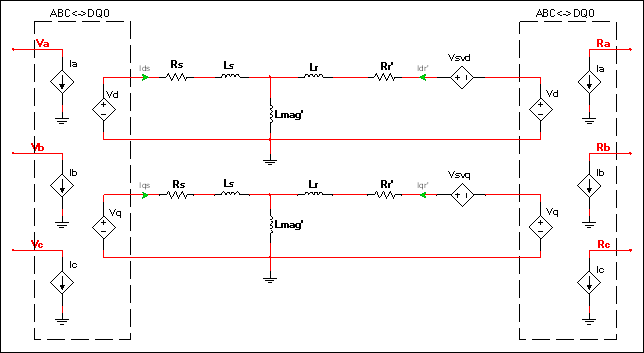OK

# Induction machine wound model

The model is based on the popular DQ0 modeling topology and is set in the stationary reference frame. The model is identical to the Induction machine squirrel cage except that the rotor windings are open-circuited.

Therefore the machine’s electrical system is slightly different and the rotor windings are exposed and provide additional connections.The element equations and the electromagnetic torque equation are identical to those shown for the Induction machine squirrel cage model.

Parameter Description
Stator leakage inductance Leakage inductance of the stationary portion of the motor.
Stator resistance Rs in the diagram of the component’s electrical system.
Rotor leakage inductance Rotor leakage inductance referred to the stator. Lr' in the Vsvd and Vsvq equations in <a href="/help/components/induction-machine-squirrel-cage/induction-machine-squirrel-cage-model/">Induction machine squirrel cage model</a>.
Rotor resistance Rotor resistance referred to the stator. Rr' in the diagram of the component’s electrical system.
Magnetizing inductance Magnetizing inductance referred to the stator. Lmag' in the Vsvd and Vsvq equations, found in <a href="/help/components/induction-machine-squirrel-cage/induction-machine-squirrel-cage-model/">Induction machine squirrel cage model</a>.
Number of pole pairs P in the equation for generated electromagnetic torque, found in <a href="/help/components/induction-machine-squirrel-cage/induction-machine-squirrel-cage-model/">Induction machine squirrel cage model</a>.
Shaft inertia J<sub>rotor</sub> on the machine model diagram in <a href="/help/components/machine-modeling/">Machine modeling</a>.
Shaft friction This is F<sub>rotor</sub> on the machine model diagram in <a href="/help/components/machine-modeling/">Machine modeling</a>.
Initial angular speed Rotational measurement of the shaft angle in rad/s at the start of the simulation.